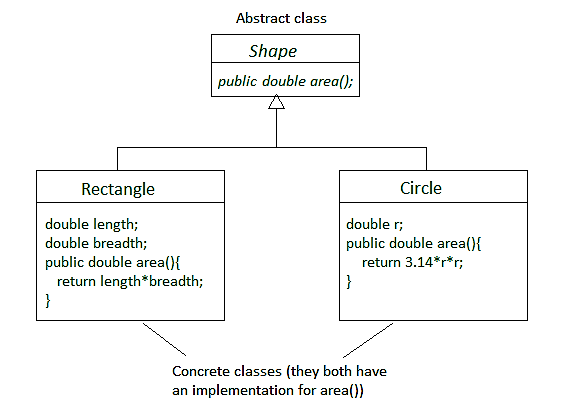# Concrete class in Java

A concrete class is a class that has an implementation for all of its methods. They cannot have any unimplemented methods. It can also extend an abstract class or implement an interface as long as it implements all their methods. It is a complete class and can be instantiated.

In other words, we can say that any class which is not abstract is a concrete class.

Necessary condition for a concrete class: There must be an implementation for each and every method.

Example: The image below shows three classes Shape, Rectangle and Circle. Shape is abstract whereas Rectangle and Circle are concrete and inherit Shape. This is because Rectangle and Circle implement area() method.Example 1: The below code shows a simple concrete class:

 `// Java program to illustrate concrete class ` ` `  `// Concrete Class ` `class` `Main { ` ` `  `    ``// this method calculates ` `    ``// product of two numbers ` `    ``static` `int` `product(``int` `a, ``int` `b) ` `    ``{ ` `        ``return` `a * b; ` `    ``} ` ` `  `    ``// this method calculates ` `    ``// sum of two numbers ` `    ``static` `int` `sum(``int` `a, ``int` `b) ` `    ``{ ` `        ``return` `a + b; ` `    ``} ` ` `  `    ``// main method ` `    ``public` `static` `void` `main(String args[]) ` `    ``{ ` `        ``int` `p = product(``5``, ``10``); ` `        ``int` `s = sum(``5``, ``10``); ` ` `  `        ``// print product ` `        ``System.out.println(``"Product: "` `+ p); ` ` `  `        ``// print sum ` `        ``System.out.println(``"Sum: "` `+ s); ` `    ``} ` `} `

Output:

```Product: 50
Sum: 15
```

Example 2: The code below illustrates a concrete class which extends an abstract class. The method product() in interface X is implemented by class Product but it does not implement method sum(), therefore it has to be abstract. Whereas class Main implements the unimplemented method sum(), therefore there are no unimplemented methods. Hence, it is a concrete class.

 `// Java program to illustrate concrete class ` ` `  `// This is an interface ` `interface` `X { ` `    ``int` `product(``int` `a, ``int` `b); ` `    ``int` `sum(``int` `a, ``int` `b); ` `} ` ` `  `// This is an abstract class ` `abstract` `class` `Product ``implements` `X { ` ` `  `    ``// this method calculates ` `    ``// product of two numbers ` `    ``public` `int` `product(``int` `a, ``int` `b) ` `    ``{ ` `        ``return` `a * b; ` `    ``} ` `} ` ` `  `// This is a concrete class that implements ` `class` `Main ``extends` `Product { ` ` `  `    ``// this method calculates ` `    ``// sum of two numbers ` `    ``public` `int` `sum(``int` `a, ``int` `b) ` `    ``{ ` `        ``return` `a + b; ` `    ``} ` ` `  `    ``// main method ` `    ``public` `static` `void` `main(String args[]) ` `    ``{ ` `        ``Main ob = ``new` `Main(); ` `        ``int` `p = ob.product(``5``, ``10``); ` `        ``int` `s = ob.sum(``5``, ``10``); ` ` `  `        ``// print product ` `        ``System.out.println(``"Product: "` `+ p); ` ` `  `        ``// print sum ` `        ``System.out.println(``"Sum: "` `+ s); ` `    ``} ` `} `

Output:

```Product: 50
Sum: 15
```

My Personal Notes arrow_drop_upCheck out this Author's contributed articles.

If you like GeeksforGeeks and would like to contribute, you can also write an article using contribute.geeksforgeeks.org or mail your article to contribute@geeksforgeeks.org. See your article appearing on the GeeksforGeeks main page and help other Geeks.

Please Improve this article if you find anything incorrect by clicking on the "Improve Article" button below.

Article Tags :
Practice Tags :

2

Please write to us at contribute@geeksforgeeks.org to report any issue with the above content.